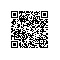# Java输入输出流和文件操作

## 操作系统中的文件和目录概念

### 文件的存取方式

①顺序存取是指按记录顺序进行读/写操作的存取方式。
②随机存取是指按记录序号进行读/写操作的存取方式。
③索引存取是基于索引文件的存取方法。由于文件中的记录不按它在文件中的位置，而按它的记录键来编址，所以用户提供给操作系统记录键后就可查找到所需记录。

### 文件的使用方式

①操作接口：操作系统将其功能和服务以操作命令形式提供给用户，用户以手动方式对文件系统等进行操作，实现人机交互功能。
②应用程序接口：操作系统将其功能和服务以系统调用（system call）形式提供给应用程序。

## 流的概念

### Java的流类和文件类

①字节流以字节为单位读/写流，用于传输非字符数据，如整数、浮点数，对象等。InputStream和OutputStream是字节输入/输出流的根类。
File文件类记载文件属性信息，RandomAccessFile随机存取文件类以随机存取方式进行文件读/写操作。

## 示例

import java.io.FileInputStream;
import java.io.FileOutputStream;

public class Main {
public static void main(String[] args) throws Exception{
String filename = "a.txt";
FileOutputStream fout = new FileOutputStream(filename);
fout.write(-1);
fout.close();

FileInputStream fin = new FileInputStream(filename);
int i;
while ((i = fin.read()) != -1){
System.out.print(i + " ");
}
fin.close();
}
}

255

public class Main {
public static void main(String[] args) throws Exception{
String filename = "a.txt";
FileOutputStream fout = new FileOutputStream(filename);
int value = -128;
fout.write(value>>>24);
fout.write(value>>>16);
fout.write(value>>>8);
fout.write(value);
fout.close();

FileInputStream fin = new FileInputStream(filename);
while ((value = fin.read()) != -1){
int temp;
for (int i = 0; i < 3 && (temp = fin.read()) != -1; i++){
value = value << 8 | temp;
}
System.out.println(value + " ");
}
fin.close();
}
}

import java.io.DataInputStream;
import java.io.DataOutputStream;
import java.io.EOFException;
import java.io.FileInputStream;
import java.io.FileOutputStream;

public class Main {
public static void main(String[] args) throws Exception{
String filename = "a.txt";
FileOutputStream fout = new FileOutputStream(filename);
DataOutputStream dout = new DataOutputStream(fout);
for (int i = 0; i < 10; i++){
dout.writeInt(i);
}
dout.close();
fout.close();

FileInputStream fin = new FileInputStream(filename);
DataInputStream din = new DataInputStream(fin);
try{
while (true){
}
}catch (EOFException ex){
//ex.printStackTrace();
}finally{
din.close();
fin.close();
}
}
}

public class Main {
public static void main(String[] args) throws Exception{
String filename = "a.txt";
FileOutputStream fout = new FileOutputStream(filename);
ObjectOutputStream objout = new ObjectOutputStream(fout);
for (int i = 0; i < 10; i++){
objout.writeObject(new TreeNode((int)(Math.random() * 100)));
}
objout.close();
fout.close();

FileInputStream fin = new FileInputStream(filename);
ObjectInputStream objin = new ObjectInputStream(fin);
try{
while (true){
}
}catch (EOFException ex){

}finally{
objin.close();
fin.close();
}
}
}

import java.io.DataInputStream;
import java.io.DataOutputStream;
import java.io.PipedInputStream;
import java.io.PipedOutputStream;
import java.io.IOException;

public class Main {
private PipedInputStream pins[];
private PipedOutputStream pouts[];
public Main(int cardMax, int number) throws IOException{
this.pins = new PipedInputStream[number];
this.pouts = new PipedOutputStream[number];
for (int i = 0; i < number; i++){
this.pins[i] = new PipedInputStream();
this.pouts[i] = new PipedOutputStream(this.pins[i]);
}

for (int i = 0; i < number; i++){
}
}

public static void main(String[] args) throws Exception{
new Main(52, 4);
}
}

private PipedOutputStream pouts[];
private int cardMax;
this.pouts = pouts;
this.cardMax = cardMax;
}

public void run(){
DataOutputStream douts[] = new DataOutputStream[this.pouts.length];
for (int i = 0; i < douts.length; i++){
douts[i] = new DataOutputStream(this.pouts[i]);
}
try{
int value = 1;
while (value <= this.cardMax){
for (int i = 0; value <= this.cardMax && i < douts.length; i++){
douts[i].writeInt(value++);
}
}
for (int i = 0; i < douts.length; i++){
douts[i].close();
this.pouts[i].close();
}
}catch(IOException ex){ ex.printStackTrace();}
}
}

private PipedInputStream pin;
private String name;
this.pin = pin;
this.name = name;
}

public void run(){
DataInputStream din = new DataInputStream(this.pin);
while (true){
try{
}
catch(IOException ex) { break; }
catch(InterruptedException ex) {}
}
try{
din.close();
this.pin.close();
}catch(IOException ex) {}
}
}

public class Main {
public static void main(String[] args) throws Exception{
String filename = "b.txt";
try{
FileWriter fw = new FileWriter("c.txt");
String line;
while ((line = br.readLine()) != null){
fw.write(line);
fw.write('\n');
}
br.close();
fr.close();
fw.close();
}catch(IOException ex){}
}
}

RandomAccessFile随机存取文件类以随机存取方式进行文件读/写操作，对一个文件可以同时进行既读又写的操作；在文件指定位置读取或者写入基本数据类型。使用钉钉扫一扫加入圈子
+ 订阅